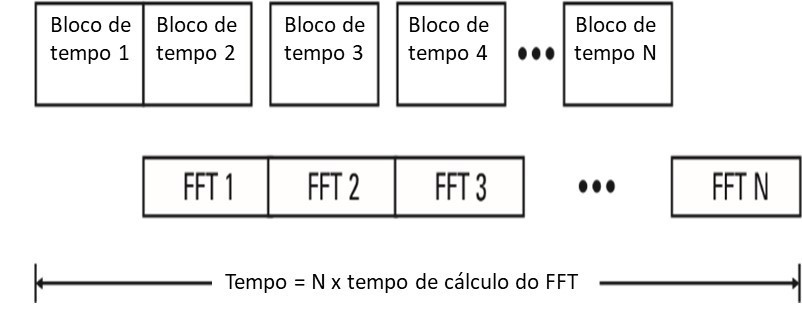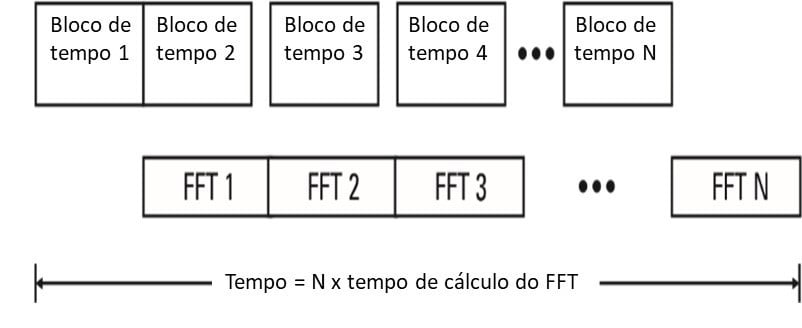# Vibration analyzer 7 – real-time bandwidth

## Vibration analyzer 7 – real-time bandwidth

Then, on vibration analyzer 7, we introduce the topic of bandwidth in real time, a vibration analyzer. Like this, this article is part of a series of articles, that explain how a vibration analyzer works. De facto, when we do Vibration Analysis, we need to understand how an analyzer works. Therefore, here we present the concepts of digital signal analysis, implemented in an FFT analyzer. actually, we always present them from the user's point of view, so that they are easy to understand. so that they are easy to understand predictive maintenance. Then, no link, we can see a range of Vibration analyzers provided by D4VIB. Then, on vibration analyzer 7, we present the content of this series of articles.

## 7 – What is real-time bandwidth

### 7.1 What time block memory is and what it is for

actually, so far, it has been ignored that, after acquiring the time block, the analyzer calculates the FFT spectrum. Furthermore, you have to take into account that it takes some time to make this calculation. In fact, we could continue to ignore it, if the FFT spectrum was calculated, in less time than the duration of a block. De facto, in order for this to be possible, we add, to the analyzer block diagram, a memory to store the time block data. Like this, this memory is before the calculation processor. Then, we see that this allows us to calculate the frequency spectrum, from the previous time block, while acquiring the current time block. Thus, we were able to increase the speed of the analyzer.Figure 7.1. As mentioned, here we see the block diagram where a memory was added to store the samples of the time block, before calculation. In this way the acquisition of a block and the FFT calculation, take place in parallel.

### 7.2 – What is real-time operation

Therefore, the analyzer is said to be operate in real time, I know we can calculate the FFT, before the time block memory fills up.Figure 7.2. actually, here we see the condition in which it is said that we are operating in real time. De facto, in this conditions, where the time for calculating the spectrum is equal to or less, to the acquisition time of a block of time, the analyzer is said to be working in real time.

### 7.3 – The operation that is not in real time

Then, let's look at what happens when the FFT calculation time, lasts longer than the acquisition of a block of time. In these circumstances, although the memory of the time block is full, the last FFT calculation has not been completed. Like this, while the calculation does not end, time block data sampling is stopped. Then, when the FFT calculation is complete, the memory can transfer the new block of time to the FFT calculation processor. In its turn, when this is finished, memory resumes acquiring samples for a new block of time. Thus, this means that some input samples are lost. Consequently, that's why, it is said that one is not operate in real.Figure 7.3. As stated, here we see the scheme of the operation in real time, due to the calculation time being longer than the acquisition time of blocks. In addition to this, it should also be remembered that the duration of the time block is not constant. De facto, this is deliberately varied, in order to change the frequency range of the spectrum. actually, as we know, the time block duration is shorter, for higher frequency ranges. Therefore, as we increase the frequency range of the analyzer, we come to a situation where, the duration of the block of time is equal to the calculation time of the FFT. Like this, this frequency value, is designated the width of real time band. Thus, the analyzer does not lose any samples of the waveform, for frequency ranges equal to or below, to real-time bandwidth.

### 7.4 How relevant is real-time bandwidth na vibration analyzer

Then, to get a sense of the relevance of real-time processing, we see some typical situations.

#### 7.4.1 What, on a machine, observe the speed variation

actually, 0 what do we want, when we look at the spectrum of a machine that is varying in speed? In this situation, de facto, it is necessary to be able to observe the successive changes in the spectrum, in order to understand what is happening. Thus, we designate this situation psychological real time. Certainly, the acquisition of a new spectrum every few tenths of a second, is fast enough to allow us to observe variations in what we consider to be in real time. However, if the machine speed variation time is long, the speed of the analyzer is irrelevant. De facto, one has to wait for the machine to vary its condition, before the spectrum is interesting. In these circumstances, no matter how many spectra are generated in that time.

#### 7.4.2 – What is the influence on the average RMS na vibration analyzer

##### 7.4.2.1 Like this, what are the conditions under which real-time bandwidth is important?
A second place, another case of interest, consists of the measures that require the realization of RMS averages. In these circumstances, we may be interested in finding the average of a signal, when it is always varying. On the one hand, there is no requirement, in the execution of averages, that the blocks of time acquired, must be consecutive, no gaps. Therefore, in these circumstances, low bandwidth in real time, does not affect the accuracy of the results of the averages. On the contrary, real-time bandwidth, affects the speed at which the RMS average can be made. De facto, in the figure 7.5, we see that, for a frequency above the real-time bandwidth, the time to complete the average of N blocks, depends only on the time to calculate the N transformed FFT. Like this, instead of continually reducing the time to average, as we increase the frequency range, we arrive at a fixed time to calculate the N averages.Figure 7.4. - As mentioned, in this figure we see the total execution time of N average RMS in a vibration analyzer Therefore, a small bandwidth in real time is just a problem when running RMS averages, when the frequency ranges used are high. In this way it is evident that the small bandwidth, In real time, it is also a problem when executing a large number of averages.
##### 7.4.2.2 actually, what is the importance of the processor in the speed with which the averages are calculated?
In this conditions, we have to wait longer for the completion of the execution of the averages. De facto, greater real-time bandwidth, require faster calculations and, therefore, a better processor. actually, it is worth noting in the case of the average RMS, greater real-time bandwidth gives slightly faster measurements. Besides that, it should be noted that this is very relevant, when many measurements are taken consecutively. actually, this is the case in which the inspection work is en route, where it is common to measure hundreds of points.

#### 7.4.3 – What is the influence on transient vibration events

Then, we see that the analysis of transient events, is the last case of interest in determining the necessary bandwidth, In real time. On the one hand, the FFT calculation time is of little interest, when the entire transient falls within the duration of the time block. In these conditions, after the block is acquired, the time the analyzer, calcula o FFT. Thus, the time to calculate the spectrum is not important. On the contrary, if the transient event contains high frequency energy and lasts longer than the time block, then the FFT calculation speed, it's critical. Then, as shown in Figure 7.6 b), some of the transients are not analyzed, if the calculation time exceeds the duration of the time block.Figure 7.5 As mentioned, in this figure, you can see the operation in real time.

#### 7.4.4 – What is the Cascada graph on a vibration analyzer

On the other hand, it must also be borne in mind that there must be a way to measure and present the results, that allows you to see the multiple events. actually, if each spectrum is erased there is a measure that a new one is obtained, not final, information was lost about what happened. Then, in the figure 7.7 we see a graph in Cascada. Like this, this presentation, is a way of seeing multiple successive spectra of transient events. actually, how do you see, with this type of chart, this occurs without loss of information.Figure 7.6. As mentioned, here we see a cascade of spectra, consecutively measured when starting a machine.